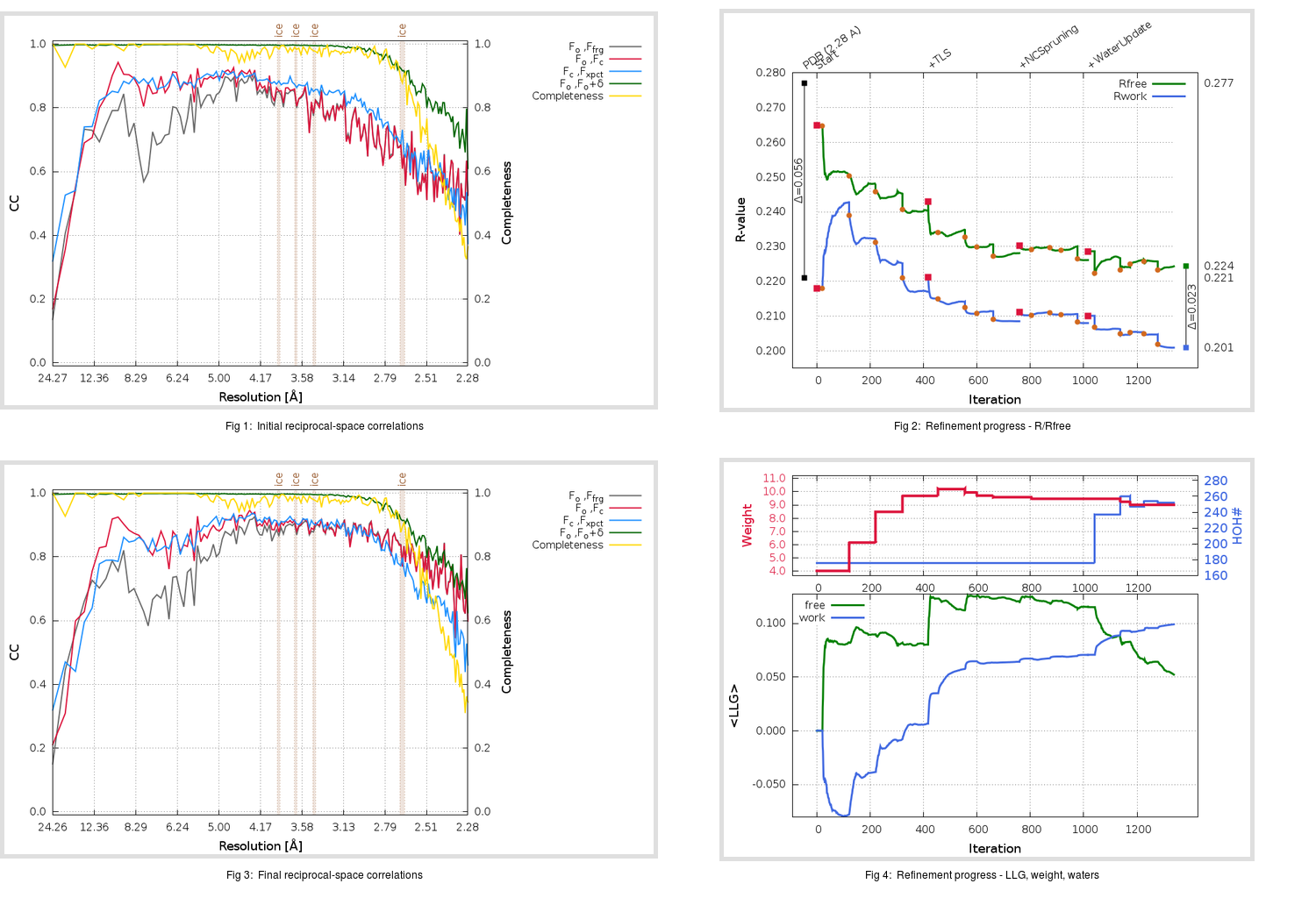Content:

```    Diffraction limits & principal axes of ellipsoid fitted to diffraction cut-off surface:
2.240         1.0000   0.0000   0.0000       0.894 a* - 0.447 b*
2.240         0.0000   1.0000   0.0000       b*
2.559         0.0000   0.0000   1.0000       c*
```

## Deposited

` `
 Date deposited Date data collection Resolution R, Rfree 20060227 20051014 2.28 0.2210 0.2770

Molprobity (CCP4 7.0 version) summary:

```Ramachandran outliers =   0.47 %
favored =  92.71 %
Rotamer outliers      =   3.45 %
C-beta deviations     =     0
Clashscore            =  20.25
RMS(bonds)            =   0.0075
RMS(angles)           =   1.45
MolProbity score      =   2.67
Resolution            =   2.28
R-work                =   0.2210
R-free                =   0.2770
```

```Number of waters      =   176

<B> (all atoms) =   58.68 ( sd =   16.09 ) for       6560 non-hydrogen atoms
<B>   (protein) =   58.83 ( sd =   16.23 ) for       6384 non-hydrogen atoms
<B>     (water) =   53.50 ( sd =    8.53 ) for        176 non-hydrogen atoms
<B>    (others) =    0.00 ( sd =    0.00 ) for          0 non-hydrogen atoms

B min/max       (all non-hydrogen atoms) =   18.04 /  112.93
B min/max   (protein non-hydrogen atoms) =   18.04 /  112.93
B min/max     (water non-hydrogen atoms) =   31.24 /   77.93
B min/max     (other non-hydrogen atoms) =    0.00 /    0.00
```

## BUSTER (re-)refinement

` `

Molprobity (CCP4 7.0 version) summary:

```Ramachandran outliers =   0.47 %
favored =  95.88 %
Rotamer outliers      =   6.35 %
C-beta deviations     =     0
Clashscore            =   3.73
RMS(bonds)            =   0.0113
RMS(angles)           =   1.67
MolProbity score      =   2.06
Resolution            =   2.28
R-work                =   0.2009
R-free                =   0.2244
```

```Number of waters      =   252

<B> (all atoms) =   59.48 ( sd =   11.83 ) for       6636 non-hydrogen atoms
<B>   (protein) =   59.36 ( sd =   11.86 ) for       6384 non-hydrogen atoms
<B>     (water) =   62.31 ( sd =   10.69 ) for        252 non-hydrogen atoms
<B>    (others) =    0.00 ( sd =    0.00 ) for          0 non-hydrogen atoms

B min/max       (all non-hydrogen atoms) =   36.40 /  101.72
B min/max   (protein non-hydrogen atoms) =   36.40 /  101.72
B min/max     (water non-hydrogen atoms) =   40.21 /   93.81
B min/max     (other non-hydrogen atoms) =    0.00 /    0.00
```

Refinement progression:Results:

` `
 File Remark 2G75_aB_refine.01_04_refine.pdb.gz exact refinement commands are in header 2G75_aB_refine.01_04_refine.mtz.gz including original deposited data and several re-refinement map coefficients 2G75_aB_refine.01_04_BUSTER_model.cif.gz including any non-standard compound restraints 2G75_aB_refine.01_04_BUSTER_refln.cif.gz